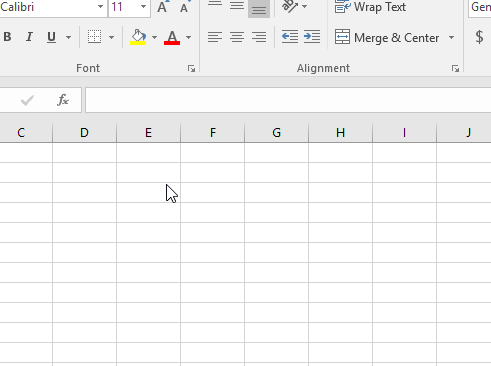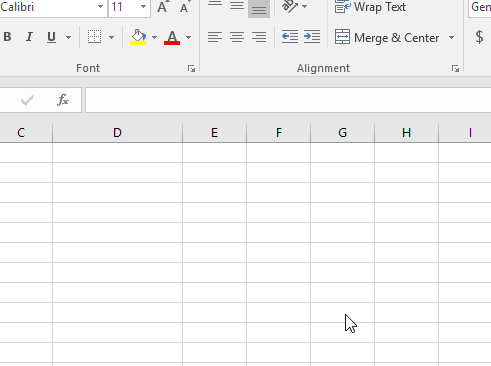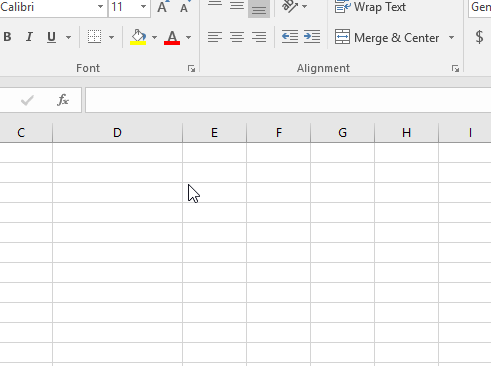# How to use TRUE function in Microsoft Excel

The TRUE function is a built-in Excel function. Excel TRUE function returns the value TRUE wherever required
Syntax:

=TRUE()

Let’s understand the Excel TRUE function.Some logic_test functions returns TRUE value when used like ISNUMBER function checks the value and returns TRUE if the value is a number.Functions use the TRUE function in formulas to provide an argument to the function.

For example: IF function returns value if condition stands true.
=IF(2<5, TRUE()) // returns TRUEAs you can see we used TRUE function as an argument to the IF function.

Hope you understood how to use TRUE function in Excel. Explore more articles on Excel logical test function here. Please feel free to state your query or feedback for the above article.

Related Articles:

How to use the Excel OR function

How to use the Excel AND Function

IF with AND Function in Excel

How to use the Excel NOT function

IF not this or that in Microsoft Excel

IF with AND and OR function in Excel

Popular Articles:

How to use the VLOOKUP Function in Excel

How to use the COUNTIF function in Excel 2016

How to Use SUMIF Function in Excel

Terms and Conditions of use

The applications/code on this site are distributed as is and without warranties or liability. In no event shall the owner of the copyrights, or the authors of the applications/code be liable for any loss of profit, any problems or any damage resulting from the use or evaluation of the applications/code.